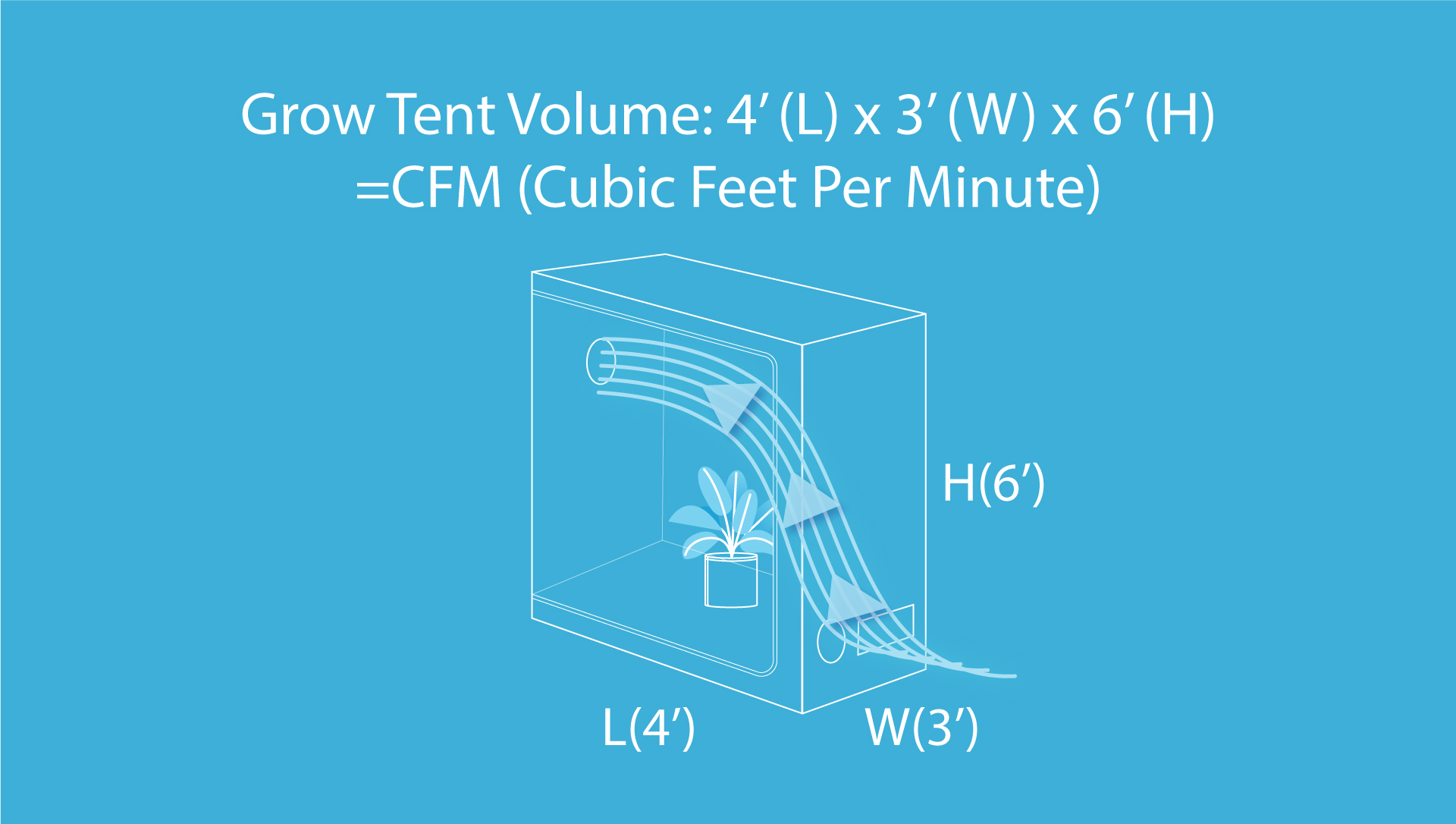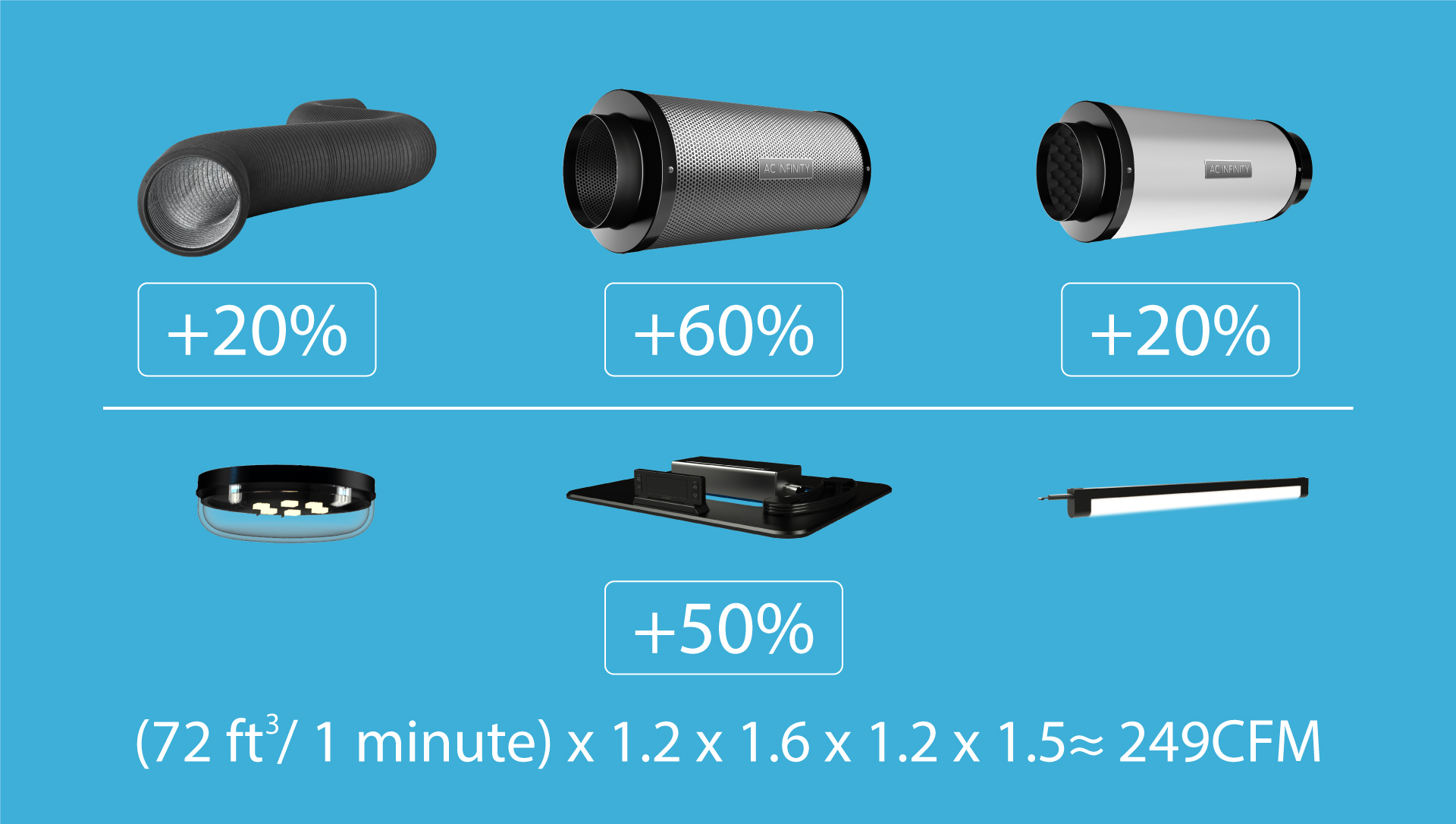If you are ventilating a grow tent, it's important to know how much air to move to provide and adequate amount of fresh air. Since it fills up the entire space, you can guess that the volume of your grow tent is equal to the amount of air that needs to be exchanged. A little more goes into the calculation if you are filling the space with accessories. So from our full grow tent ventilation guide, learn how to find the CFM you need.

## The Formula

First find its volume in cubic feet by multiplying its length by its width and height. Convert units of measurement as needed. This will equal your space’s volume, with the required airflow equal to this figure in cubic feet per minute, or CFM. Here is what the formula looks like:For example, a 48”x36”x72” grow tent converted to feet would be a 4’x3’x6’ grow tent. Multiplying the dimensions together would equal 72 ft 3. Since a grow space’s volume is also the required CFM to ventilate it every minute, this grow tent’s base CFM is 72.

## Grow Tent Accessories

The next step is to increase your base CFM by your accessories’ efficiency percentages. Adding components like ducting and carbon filters will reduce fan performance in your grow space which affects are hard your fan needs to work.

When it comes to ducting, the number and sharpness of its bends factor into the airflow resistance. This is because airflow reduces the further it must travel so the straighter the ducting route the better. The sharper the bend, the harsher the efficiency reduction; a 30° bend cuts the amount of airflow by 20% while a 90° bend diminishes airflow by 60%! Smoothing out any wrinkles can also improve fan performance and airflow.

If you are using LED grow lights, you must also account for its heat output which further increases your required CFM by up to 50%.

These factors add to your required CFM, which calls for a high performing fan in order to move the calculated airflow. Because accessories on the market can greatly vary, you can use estimated efficiency percentages depending on the type of component.

(Base CFM x Component factors) x (Grow light heat) = Required CFM

For example, our 4’x3’x6’ grow tent has a baseline CFM of 72. We multiply this figure by the efficiency percentage of each component. If we add a carbon filter (60%), ducting (~20%), and a silencer (20%) to our ventilation system, we get 166 CFM. From here, factoring in your grow light’s heat output (≤50%) will give us a required CFM of 249. Keep in mind that these percentages are not fixed and may vary. Here is the breakdown of this calculation:Multiplying your base CFM (72) by the ducting (20%), carbon filter (60%), silencer (20%), and grow light heat (50%) of your choice will yield about a required 249 CFM.